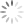# Design Guidelines for RT7302 and RT7304 PSR LED Driver

## Abstract

RT7302 and RT7304 are constant current LED drivers with active power factor correction (PFC). They support high power factor across a wide range of line voltages, and drive the converter in the quasi-resonant (QR) mode to achieve higher efficiency. By using primary side regulation (PSR), RT7302/RT7304 control the output current accurately without the need of a shunt regulator or opto-coupler at the secondary side, reducing the external component count, the cost, and the size of the driver board.

This application note presents a step by step design guideline for an isolated single stage constant current LED driver with PFC using the RT7302. The guideline can also be applied to RT7304.
The design example in this application note describes an 18W LED driver with slim form-factor, suitable for T8 LED tube applications, but the same design can also be used in LED bulb or other form factor applications.

## 1. Introduction

RT7302 and RT7304 are constant current LED drivers with active power factor correction (PFC). They support high power factor across a wide range of line voltages, and drive the converter in the quasi-resonant (QR) mode to achieve higher efficiency. By using primary side regulation (PSR), RT7302/RT7304 control the output current accurately without a shunt regulator or opto-coupler at the secondary side, reducing the external component count, the cost, and the size of the LED driver board.
RT7304 embeds comprehensive protection functions for robust designs, including LED open circuit protection, LED short circuit protection, output diode short circuit protection, VDD under voltage lockout (UVLO), VDD over-voltage protection (VDD OVP), over-temperature protection (OTP), and cycle-by-cycle current limitation. RT7304 is available in a cost effective SOT-23-6 package.
RT7302 has the same basic functionality as RT7304, but integrates more features, including fast startup via high voltage pin, PWM dimming, and input voltage feed-forward compensation. RT7302 is available with SOP-8 package.

This application note presents a step by step design guideline for an isolated single stage constant current LED driver with PFC using the RT7302. The design guideline can also be applied to RT7304.
The design example in this application note is an 18W LED driver with slim form-factor, suitable for T8 LED tube applications.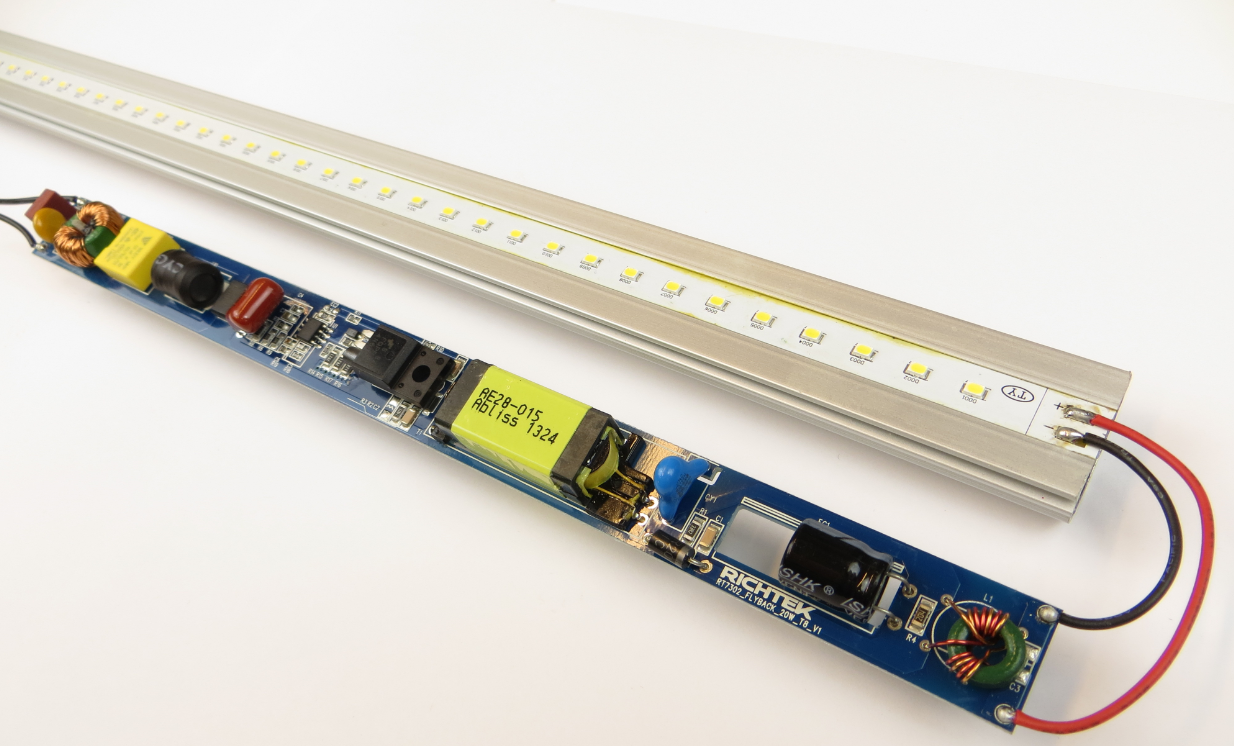Figure 1. Picture of the 18W evaluation board with a typical T8 LED assembly

## 2. RT7302 Basic Operation

Figure 2 shows RT7302 in a typical flyback converter topology with input voltage (Vin).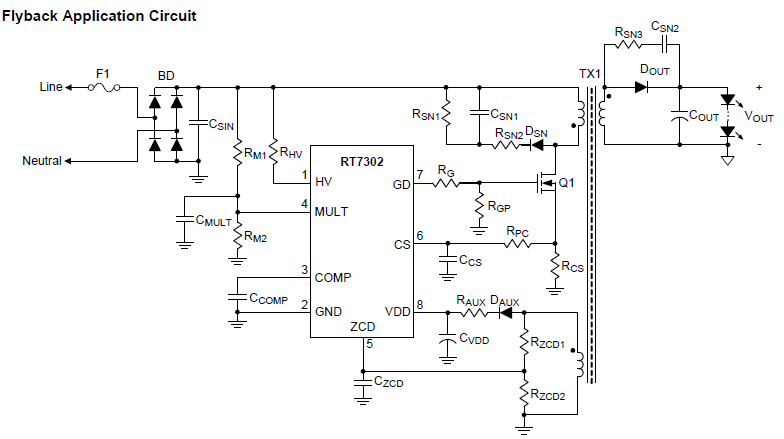Figure 2

When main switch Q1 is turned on with a fixed on-time ton, the peak current IL_pk of the magnetic inductor Lm can be calculated by the following equation: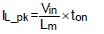If the input voltage is the output voltage of the full-bridge rectifier with sinusoidal input voltage Vin_pk·sin(θ), the inductor peak current IL_pk can be expressed as the following equation: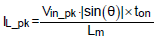When the converter operates in critical-conduction mode (CRM) with constant on-time control, the envelope of the peak inductor current will follow the input voltage waveform in-phase. Thus, high power factor can be achieved. The minimum on time is set by the upper divider resistor of the ZCD network RZCD1.

Quasi resonant switching is achieved by sensing the auxiliary winding zero current condition and a smart internal valley detection circuit. Switch-on of the MOSFET will always happen at a valley of the resonant voltage, thereby reducing switching losses and EMI. The ZCD pin is also used to sense output OVP condition, set by RZCD2.

The primary peak current is sensed by measuring the voltage across the MOSFET source resistor via the CS pin. An internal leading edge blanking circuit removes any spikes from this signal. A current variation due to a propagation delay is compensated by the CS pin internal current source and the external series resistor RPC.

The MULT pin is used for sensing the input peak voltage, and controls the ramp for T-on generation. The line voltage sense is used as feed-forward to adjust the ramp for constant COMP voltage over line voltage. This improves the regulation, eases compensation and achieves accurate maximum power limit over the full mains range. This is especially important for full range LED driver designs.

RT7302 HV pin will quickly charge the capacitor connected to the VDD pin during start-up. After start-up, the HV pin is disconnected, and the VDD is supplied by the auxiliary winding. This method ensures fast start-up without extra power dissipation in the bleeder resistor during normal operation.

Design Procedure:

The basic design sequence is as following:

Define Input and output conditions → Calculate input power → Transformer design, Calculate N ratio, Primary inductance, Primary and secondary winding turns → Current sense resistor (RCS), bridge rectifier, MOSFET parameters, Output diode parameters → Minimum ton setting (RZCD1) → OVP setting (RZCD2) → Propagation delay setting (RPC) → Feed-forward compensation (RM1, RM2)

The RT7302 design tool can be used to quickly determine the component values. Chapter 3 contains a detailed step by step design description for the 18W reference design.

## 3. Design of an 18W LED Driver for T8 Applications

The LED driver example for this section is the 18W T8 LED driver evaluation board, see Figure 3.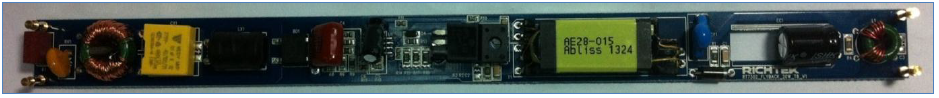Figure 3. The board measures 230x18x10mm and will fit in most narrow tube T8 housing behind the LED board.

Requirement specification for this design:

• Input range: 90V ~ 264Vac
• LED load: 45V / 400mA
• Efficiency >85% at 120V / 230Vac
• PF: > 0.95 and THDi < 15% (meet IEC61000-3-2 class C & D)

Step 1. Input and Output Conditions

The input and output conditions are listed as follows:
maximum AC input voltage Vac_max, 264

Vac
minimum AC input voltage Vac_min, 90Vac
line frequency fline, 50Hz / 60Hz
average output current IO, 400mA
minimum average output voltage Vo_min, 43V
maximum average output voltage Vo_max, 47V
LED string is using 14 high power LEDs with total dynamic resistance of 14Ω

Estimated maximum average input power Pin_max_est can be expressed as: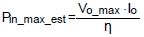where η is the estimated efficiency.
The efficiency is estimated at 85%, the input power will become : 47*0.4/0.85 = 22.12W.

Estimated peak current transfer ratio of the transformer (CTRTX1) can be expressed as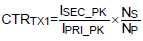where ISEC_pk is the peak current of secondary side, IPRI_pk is the peak current of the primary side, NS is the turn’s number of the secondary winding, and NP is the turn’s number of the primary winding. CTRTX1 can be estimated to be 0.9.

The reflected output voltage Vro can be express as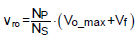where Vf is the forward voltage of output diode. Vro is recommended to be within 95 ~125V.
In the example: Set Vro = 125V.

Min. VDD supply voltage at max. output voltage VDD_Vomax_min can be derived as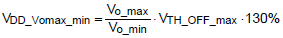where VTH_OFF is the falling under voltage lockout (UVLO) threshold voltage of the controller.

VDD supply voltage at max. output voltage VDD_max must be within VDD_Vomax_min ~ VDD_OVP_min.

In the example:
VO_max = 47V, VO_min = 43V, VTH_OFF_max = 10V, VDD_Vomax_min= 14.2V
Set VDD_max = 20V.

Output Capacitor COUT Calculation:

The output capacitor value will determine the amount of voltage ripple on the LED string. This voltage ripple, together with the dynamic resistance of the LED string will determine the current ripple through the LED string and this will cause 100Hz or 120Hz light flicker.

In this example the maximum allowed LED current ripple amplitude is set at 340mApp for a ripple percentage of 42%. The LED string uses 14 LEDs and has total dynamic resistance of 14Ω: VOUT ripple = 0.34A*14 Ω = 4.76Vpp. The transformer secondary winding current can estimated having a low frequency ripple of double the line frequency and low frequency peak to peak amplitude of double the average output current. The output capacitor value can now be calculated: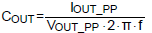where IOUT_PP is 2x the average LED current, and VOUT_PP is the allowed AC output voltage ripple and f is double line frequency. Calculating for 50Hz line frequency: COUT = 2*0.4/(4.76*2*π*100) = 267µF. For less LED current ripple, the COUT value needs to be increased. But when LED strings with higher dynamic resistance are used, the COUT value can be reduced.

Step 2. Transformer Design

Ideal turn’s ratio of primary to secondary windings can be expressed as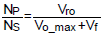In the example:
Vro = 125V, VO_max = 47V, Vf = 0.7V, NP/NS= 2.62
Ideal turn’s ratio of secondary to auxiliary windings can be expressed as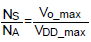In the example:
VO_max = 47V, VDD_max = 20V, NS/NA = 2.35
The maximum on time of the MOSFET ton_max can be expressed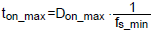in which fs_min is the minimum switching frequency.
The duty ratio of the MOSFET Don can be expressed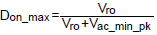The primary-side inductance Lm can be derived as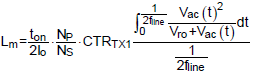Thus, Lm can be obtained after the minimum switching frequency fs_min is determined.

In the example:
Set fs_min = 54kHz,
Vro = 125V, Vac_min_pk = 127V,
Obtain ton_max = 8.68μs and Lm = 899μH
The minimum number of turns for the transformer primary side to avoid the core saturation is given by: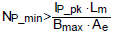where Ae is the cross-sectional area of the core in m2, and Bmax is the maximum flux density in Gauss.

In the example:
IP_pk = 1.23A, Lm = 899μH, EDR-28 core is chosen and its Ae = 88m2.
Set Bmax = 2950 Gauss. Obtain NP_min > 42.5 turns.
Now all the parameters of transformer are determined, including NP_min, NP/NS, NS/NA and Lm.
NP = 43T, NS = 43/2.62 = 16.4T, choose 16T, NA = 16/2.35 = 6.8T, choose 7T.

Step 3. Current Sense Resistor Determination

Current sense resistor RCS can be determined as the following equation: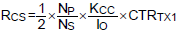where KCC is a reference in the controller.

In the example:
Actual NP/NS = 2.69, KCC = 0.25, IO = 0.4A, CTRTX1= 0.9,
The current sense resistor RCS will become (1/2)*2.69*(0.25/0.4)*0.9 = 0.79 Ω.

Step 4. Bridge Rectifier Determination

The maximum reverse voltage of the bridge rectifier VRRM_max can be expressed as: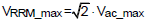The maximum forward current of the bridge rectifier IBR_max can be expressed as:In the example: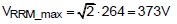IBR_max = 22.12/90 = 0.25A
A 600V / 1A diode bridge will provide sufficient de-rating, including inrush current and voltage surge.

Step 5. MOSFET Determination

The maximum drain-to-source voltage stress of the MOSFET VDS_max is given as: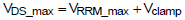in which Vclamp is the maximum voltage on the snubber and it must be higher than Vro.
The maximum drain-to-source current stress of the MOSFET IDS_max is given as: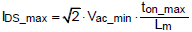In the example:
Set Vclamp = 160V
VDS_max = 373+160 = 533V : for sufficient de-rating, choose at least 650V rated MOSFET.
IDS_max = IP_pk = 1.23A : MOSFET Rdson selection depends on thermal aspects. In this small T8 design, a 4A MOSFET with Rdson of 1.8Ω was selected, which can be used without heat-sink.

Step 6. Output Diode and Auxiliary Diode Determination

The maximum reverse voltage stress of the output diode VDo_max can be expressed as: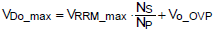where VO_OVP is the output over voltage level.
The maximum average forward current stress of the output diode IDo_max can be expressed as: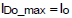In the example:
Set VO_OVP = 61V
VDo_max = 373/2.62+61 = 203V
IDo_max = IO = 0.4A
Diodes with higher current rating can be chosen for higher efficiency.

The maximum reverse voltage stress of the auxiliary diode VDa_max can be expressed as: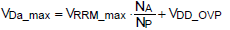where VDD_OVP is the VDD over voltage level.
The maximum average forward current stress of the output diode IDa_max can be expressed as: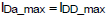in which IDD_max is the maximum supply current for the controller.

In the example:
VDD_OVP = 27V
VDa_max = 373/(2.62*2.35)+27 = 87.8V
IDa_max = IDD_max = 5mA

Step 7. Minimum On-Time Setting

RT7302 limits a minimum on-time ton_min for each switching cycle. The ton_min is a function of the sample-and-hold ZCD current IZCD_SH as following: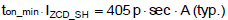IZCD_SH can be expressed as: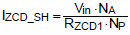Thus, RZCD1 can be determined by: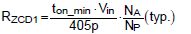In addition, the current flowing out of ZCD pin must be lower than 2.5mA (typ.). Thus, the RZCD1 is also determined by: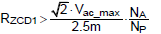In the example: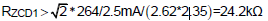Set RZCD1 =60kΩ
When Vin = 10V, ton_min = 405p*60k*(2.62*2.35)/10 = 14.9μs
In general, longer ton_min can slightly improve THDi. However, if ton_min is too long, it will induce a current resonance at Vin zero crossing, worsening THDi. Thus, ton_min can be properly defined according to the measured THDi.

Step 8. Output Over-Voltage Protection Setting

Output OVP is achieved by sensing the knee voltage on the auxiliary winging. Thus, RZCD1 and RZCD2 can be determined by the equation as: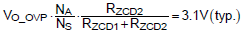In the example:
Set VO_OVP = 61V
It can be calculated that RZCD2 =7.9kΩ

Step 9. Propagation Delay Compensation Design

The VCS deviation (ΔVCS) caused by propagation delay effect can be derived as: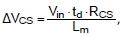in which td is the delay period which includes the propagation delay of RT7302 and the turn-off transition of the main MOSFET. The sourcing current from CS pin of RT7302 ICS can be expressed as: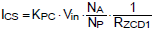where KPC is a constant value in the controller. RPC can be designed by: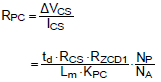td is estimated to be around 150ns

In the example:
RPC = 150n*0.74*60k/(899μ*0.02)*(2.62*2.35) = 2.3kΩ

The delay period td is varied with the parasitic capacitance of MOSFET, the gate driving capability, and the propagation delay of the controller. Thus, td cannot be estimated accurately, and RPC may need to be modified according to the measured output current. If the output current increases when Vin rises, RPC should be increased. If the output current decreases with Vin rises, RPC should be decreased.

Step 10. Feed-Forward Compensation Design (Only for RT7302)

The COMP voltage, VCOMP, can be derived from the following equations.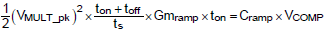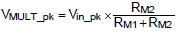VMULT_pk is the peak voltage on the MULT pin. Gmramp is the trans-conductance of the ramp generator, and its typical value is 2.5μA/V. Cramp is the capacitance of the ramp generator, and its typical value is 6.5pF. When the converter operates at CRM, (ton + toff) / tS=1. VCOMP_min is recommended be within 1.2 ~ 1.5V, and RM2 is recommended to be within 30 ~ 60kΩ. Thus, the voltage divider resistors RM1 and RM2 can be determined according to the above parameters.

In the example:
ton_max = 8.68μs.
Set VCOMP_min = 1.2V,
Obtain VMULT_pk = 0.85V.
Set RM2 = 43kΩ,
It can be calculated that RM1 = 6.4MΩ.

## 4. Design Tool Explanation

The RT7302 design tool and RT7304 design tool can be used as quick way to determine the component values. The content is similar to the step by step design as described in Chapter 3. In the design tool, users input operation parameters into "Yellow Grid". According to the key-in parameter, the design tool will automatically generate the results in Pink Grid".

Table 1 below shows the entered data and calculation results for the 18W T8 reference design.

Table 1. Design tool data

## 5. Circuit Diagram of the Evaluation Board

The circuit diagram of the evaluation board is shown in Figure 4 below.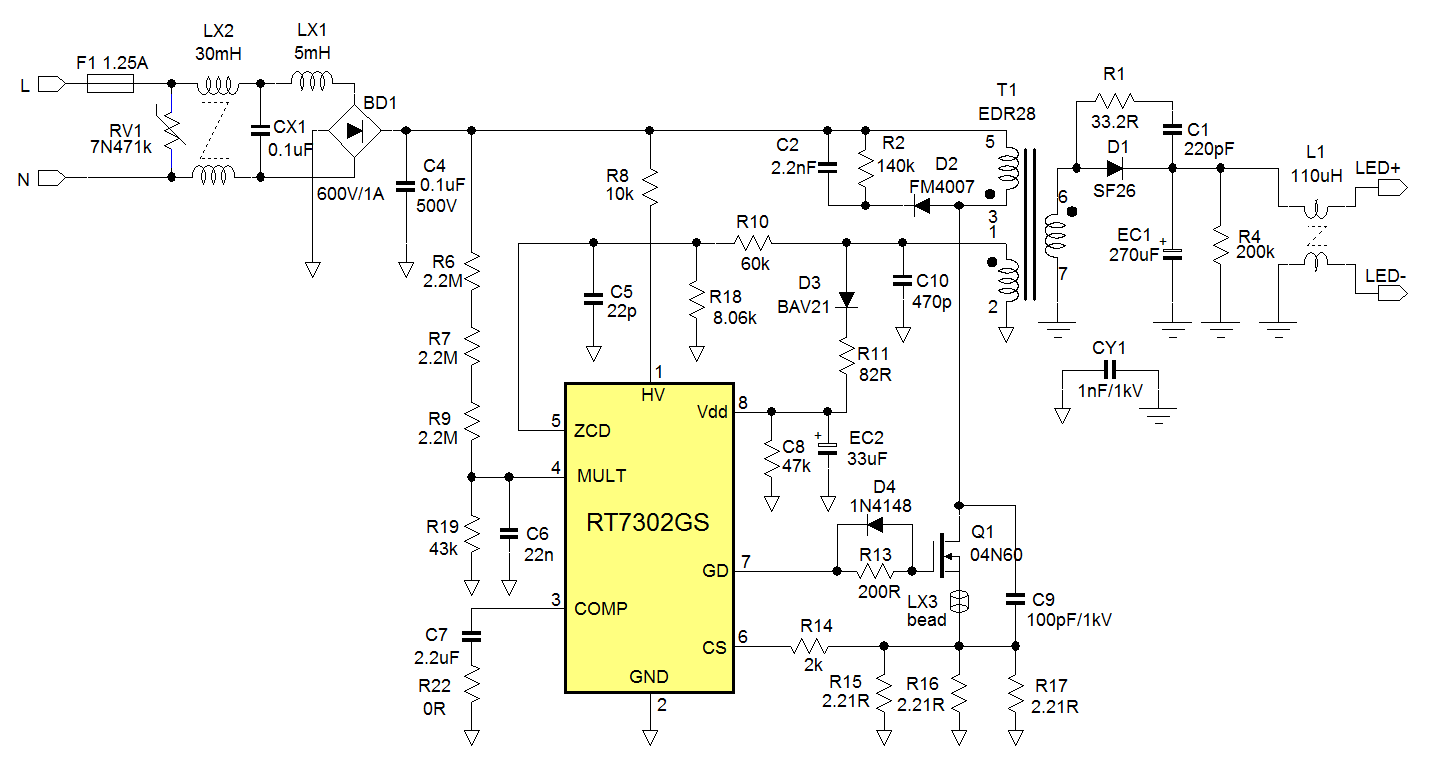Figure 4. Schematic of the 18W T8 LED driver reference design

RV1 is added for line surge protection. LX2, CX1 and LX1 are added to reduce Line conducted EMI. L1 and LX3 are added to reduce radiated EMI.

The full BOM is shown in table 2 below.

Table 2. Full BOM of the 18W LED driver reference design

 Item Quantity Reference Part/Value Type Vendor Remark 1 1 F1 T1.25A/300V SS-5F-2P Littlefuse 2 1 RV1 7N471K TVS-2P Thinking 3 1 LX2 30mH LRS-T14 Abliss T12.7*7.92*4.9(μi=10000) 4 1 CX1 0.1μF CFS-12X12 Shiny Space 5 1 LX1 5mH LDS-D9X12 Mag. layers 6 1 BD1 1A/600V DB-1A GW 7 1 C4 0.1μF/500V CFS-11X10 Murata 8 3 R6, R7, R9 2.2MΩ 0805 RALEC 9 1 R19 43kΩ 0603 RALEC 10 1 C6 22nF/50V 0603 Murata 11 1 C7 2.2μF/25V 0805 Murata 12 1 R22 0Ω 0603 RALEC 13 1 R8 10kΩ 1206 RALEC 14 1 R2 140kΩ 1206 RALEC 15 1 D2 FM4007 SOD123 Willas 16 1 C2 2.2nF/1kV 1206 Murata 17 1 R13 200Ω 0805 RALEC 18 1 D4 1N4148 SOD-123 Willas 19 1 Q1 4A/650V TO-220 IPS FTA04N65D 20 1 C9 100pF/1kV 1206 Murata 21 1 LX3 T3.5*3*1.4 --- King core On Source pin of Q1 22 3 R15, R16, R17 2.21Ω 1206 RALEC 23 1 R14 2kΩ 603 RALEC 24 1 C10 470pF/1kV 1206 Murata 25 1 CY1 1000pF/250Vac CAP-10mm Murata 26 1 R10 60kΩ 0603 RALEC 27 1 R18 8.06kΩ 0603 RALEC 28 1 C5 22pF 0603 Murata 29 1 D3 BAV21 SOD-123 Willas 30 1 R11 82Ω 0603 RALEC 31 1 EC2 33μF/50V CES-5X11 Rubycon 32 1 T1 EDR28 EDR28 Abliss 33 1 U1 RT7302 SOP-8 Richtek 34 1 D1 SF26 DO-15 Willas 35 1 R1 33Ω 1206 RALEC 36 1 C1 220pF/1kV 1206 Murata 37 1 EC1 270μF/63V CES-10X25 Rubycon 38 1 L1 110μH LR-T9 Abliss T9*5*3(μi=10000) 39 1 R4 200kΩ 1206 RALEC

Transformer design: The transformer design specification is shown in Figure 5.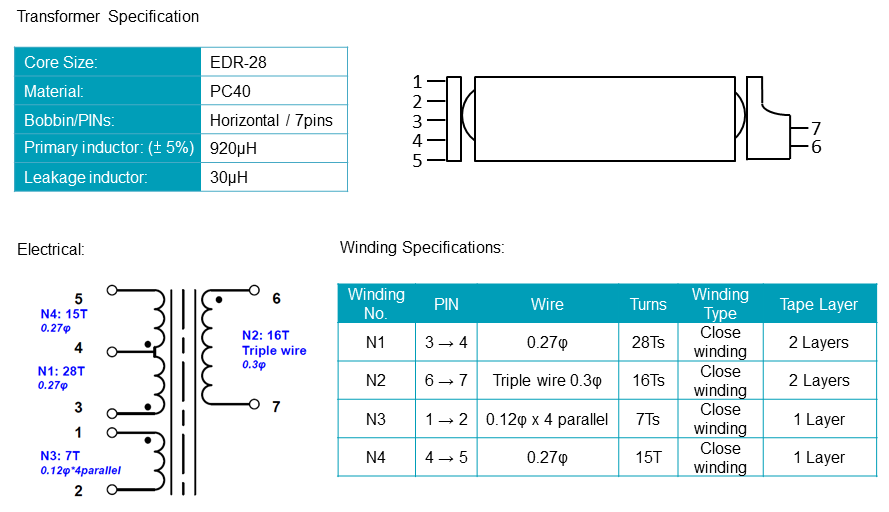Figure 5. Transformer specification

The primary side formed by sandwich structure is used to reduce the leakage inductance of the transformer, improving the efficiency and the output current regulation. To improve radiation EMI, the maximum voltage swing which is on pin 3 of the transformer, should be at the most inner side. To meet the safety standard, Triple wire at the secondary side is normally adopted for providing insulation.

## 6. Electrical Performance Measurements

Table 3 below shows the LED driver input and output parameters over the full mains voltage range.

Table 3. Performance measurements

 Frequency Vac [V] Pin [Watt] Vout [V] Iout [mA] Pout [Watt] Eff. [%] PF Value THD 60Hz 90 21.54 45.75 405 18.53 86.02% 0.9960 6.37 60Hz 100 21.24 45.78 405 18.54 87.29% 0.9960 6.68 60Hz 110 21.03 45.80 404 18.50 87.98% 0.9954 7.03 60Hz 120 20.87 45.83 403 18.47 88.50% 0.9950 7.24 60Hz 132 20.73 45.86 402 18.44 88.93% 0.9944 7.53 50Hz 180 20.60 46.00 401 18.45 89.54% 0.9908 7.51 50Hz 200 20.60 46.07 400 18.43 89.46% 0.9886 7.02 50Hz 220 20.64 46.15 400 18.46 89.44% 0.9851 6.73 50Hz 230 20.69 46.23 400 18.49 89.38% 0.9832 6.82 50Hz 240 20.75 46.31 400 18.52 89.27% 0.9811 6.99 50Hz 264 20.90 46.44 400 18.58 88.88% 0.9738 7.86

Current regulation = 1.23%
ΔEfficiency = 3.52%
Maximum PF = 0.996
Minimum PF = 0.974

As can be seen, the current line regulation is excellent. Driver efficiency meets the target easily, and Power Factor and THDi are fully in line with regulations for lighting applications.

The figures in Table 4 show voltage and current waveforms during various operation conditions:

Table 4. Measured waveforms during various operation conditions

 Start-up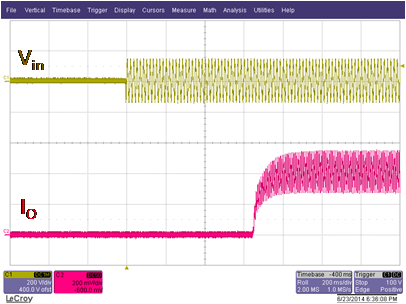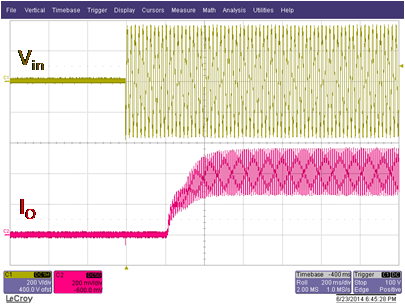Vin = 90Vac: T-start = 630msec Vin = 264Vac: T-start = 210msec Input waveform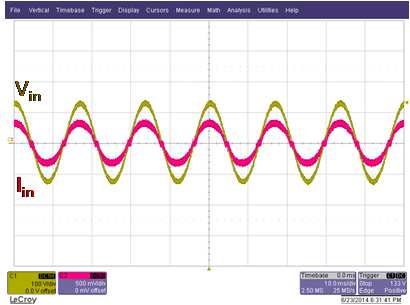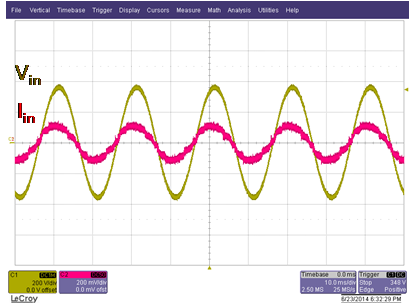Vin = 90Vac Vin = 264Vac Output waveform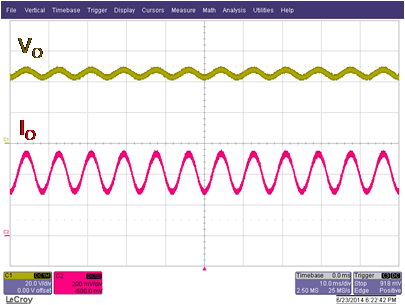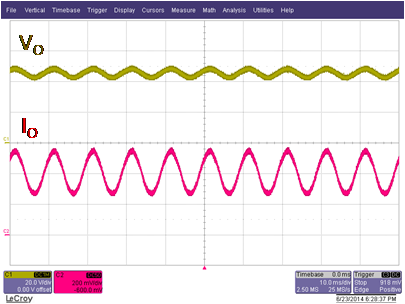Vin = 90Vac Vin = 264Vac Harmonic content of input current: (IEC61000-3-2)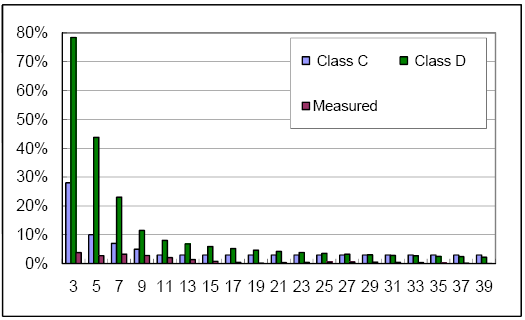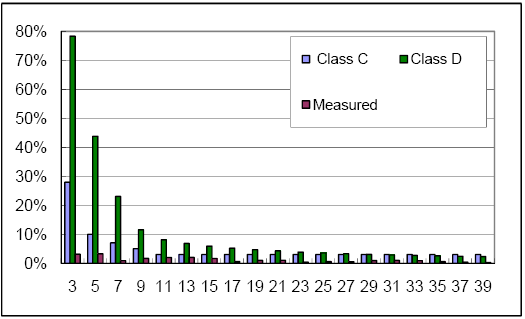Vin = 110Vac: passes Class C and D Vin = 230Vac: passes Class C and D Conduction EMI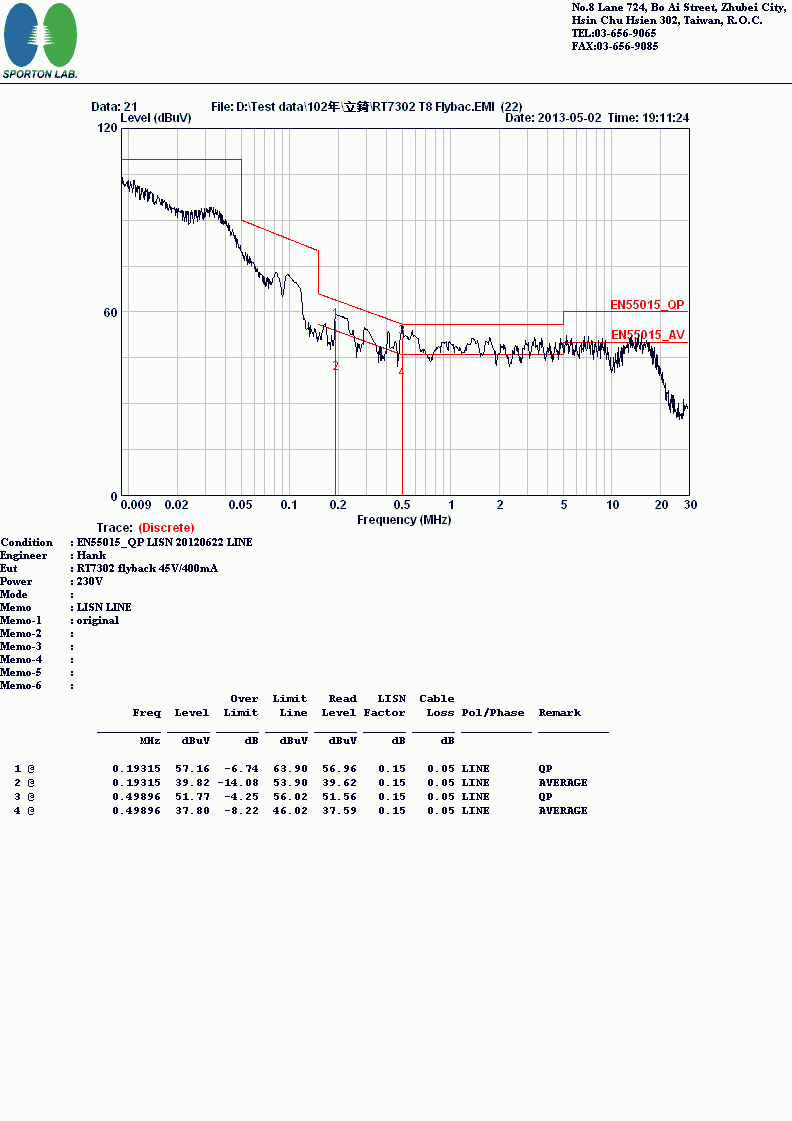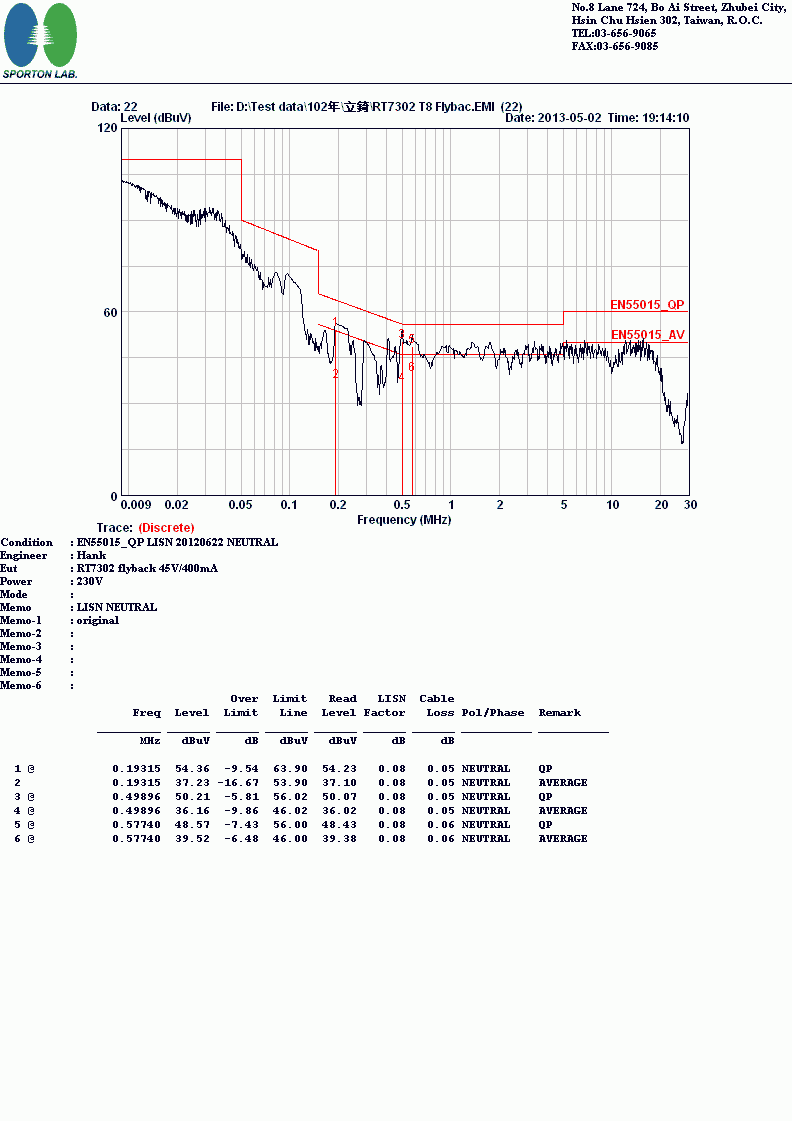Vin = 230V-L Vin = 230V-N The demo board passes conducted and radiated EMI at 120V and 230Vac

## 7. PCB Layout Information

The PCB layout of the reference design is shown in Figure 6 below. It is build from double-sided FR-4 material and uses a narrow form-factor to make it suitable to fit into narrow T8 enclosures.
To minimize EMI, current loops of the gate drive, snubber circuit, output diode and main MOSFET switching loop should be kept as small as possible. Ground of the IC, sense resistor, aux winding and Y-capacitor should all go to one central ground point on the input capacitor ground. Capacitors on the IC COMP pin, ZCD pin and MULT pin should be as close as possible to the IC.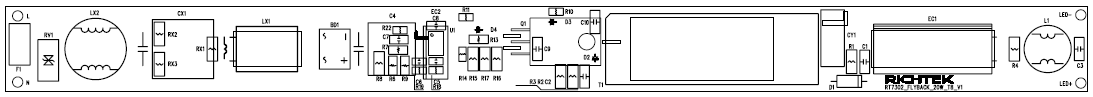Top silk (Component location)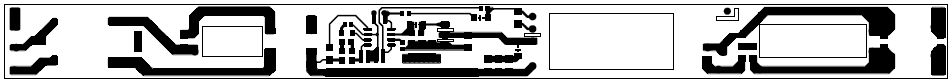Top trace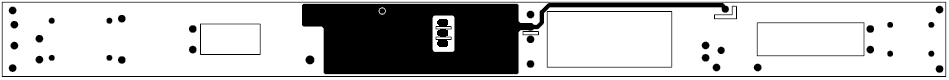Bottom trace

Figure 6. PCB Layout

## 8. Summary

With the help of this step by step design guide and the RT7302 design tool, the user is able to quickly design a LED driver that fulfills the requirements for high performance offline LED drivers. The absence of secondary side sensing greatly simplifies the mechanical design, and allows small form-factor PCB design.
When all guidelines are followed, the design should fulfill EMI and pass the surge tests. Although this reference design is for an 18W LED driver, RT7302 can be used in a wide range of LED driver designs, ranging from 8W ~ 60W.Table of Content